Ex 11.2

Chapter 11 Class 10 Constructions [Deleted]
Serial order wise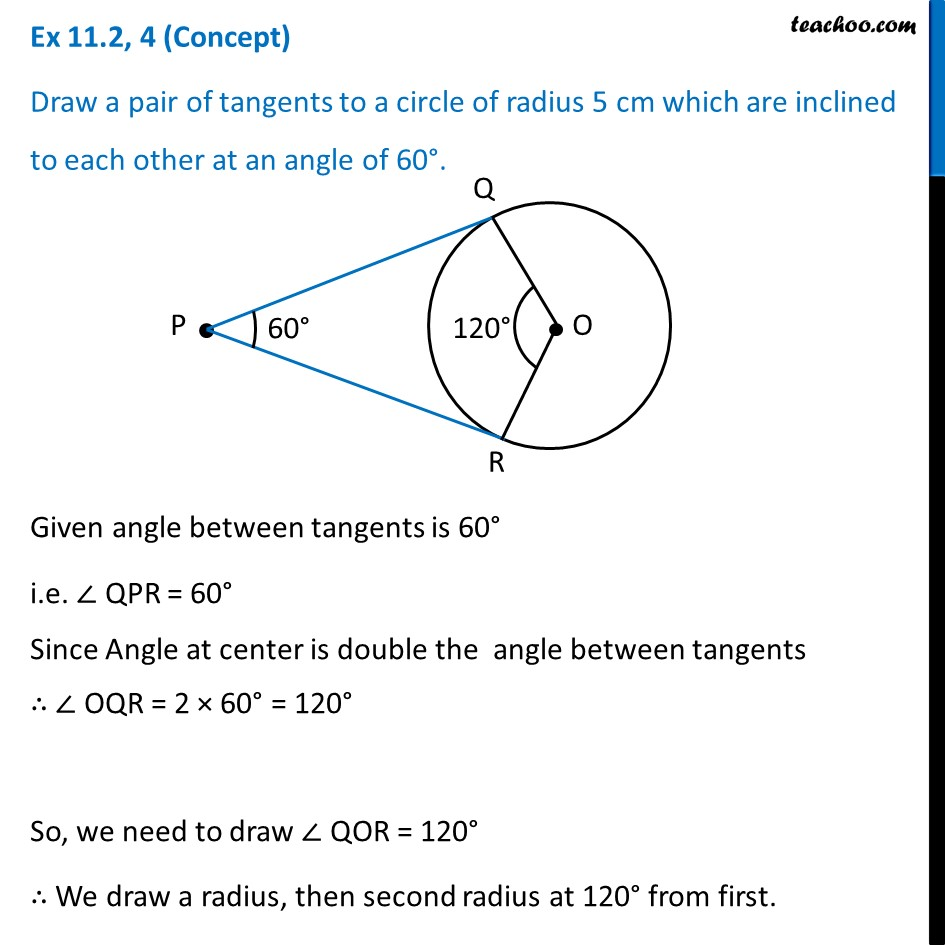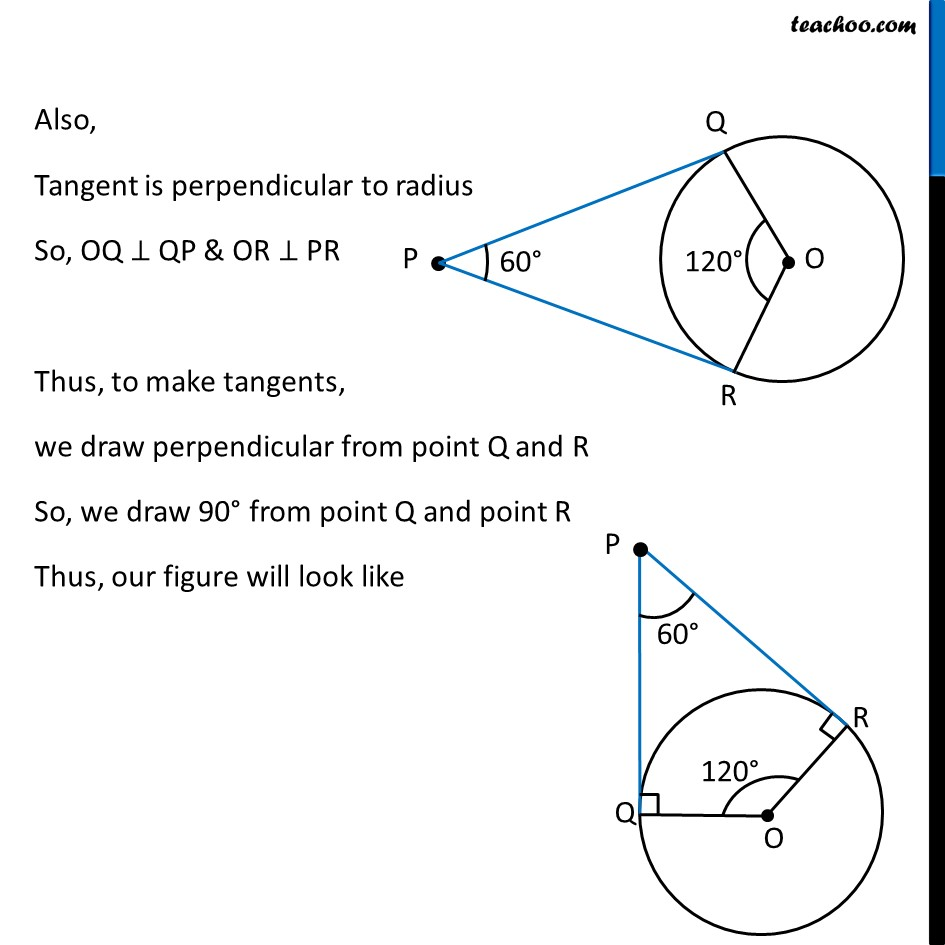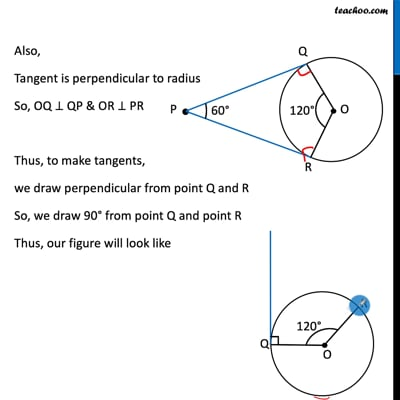This video is only available for Teachoo black users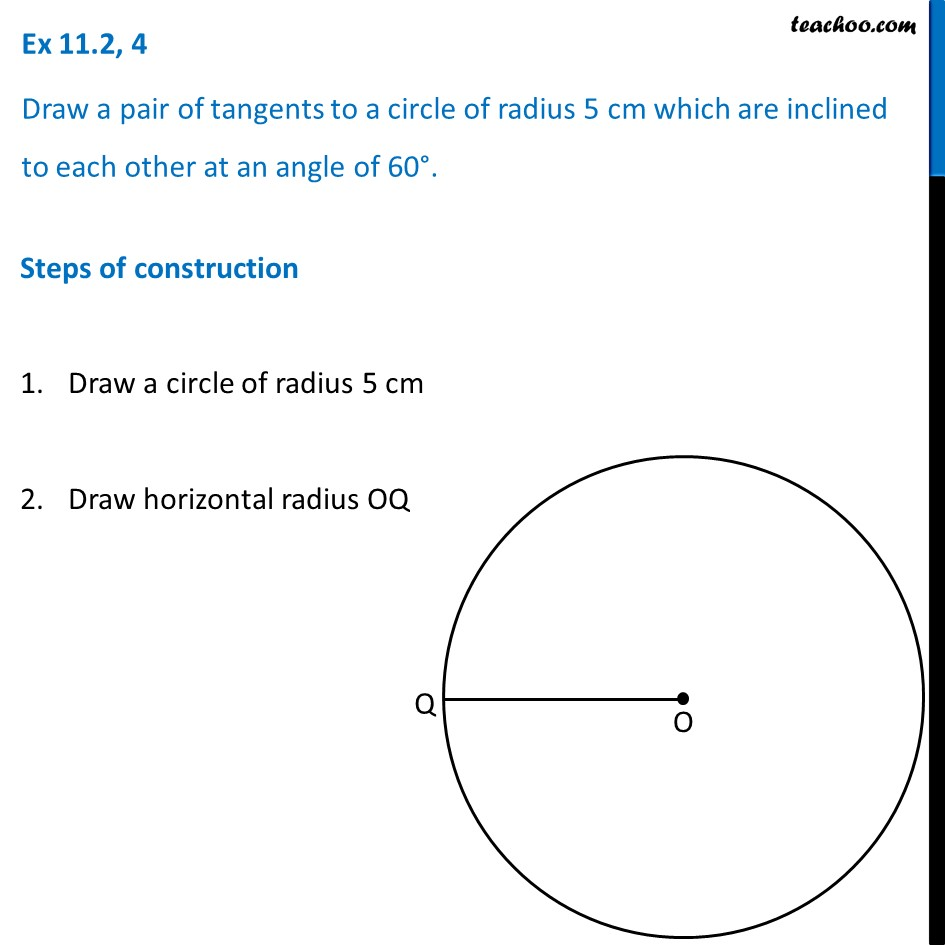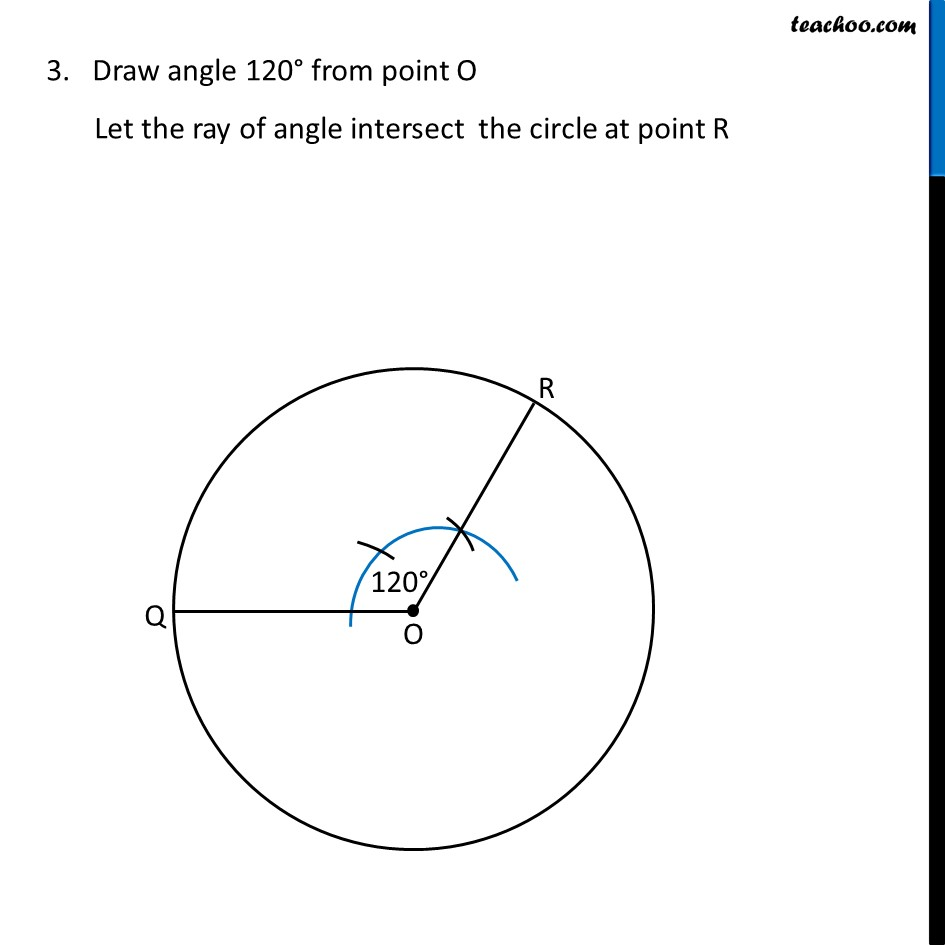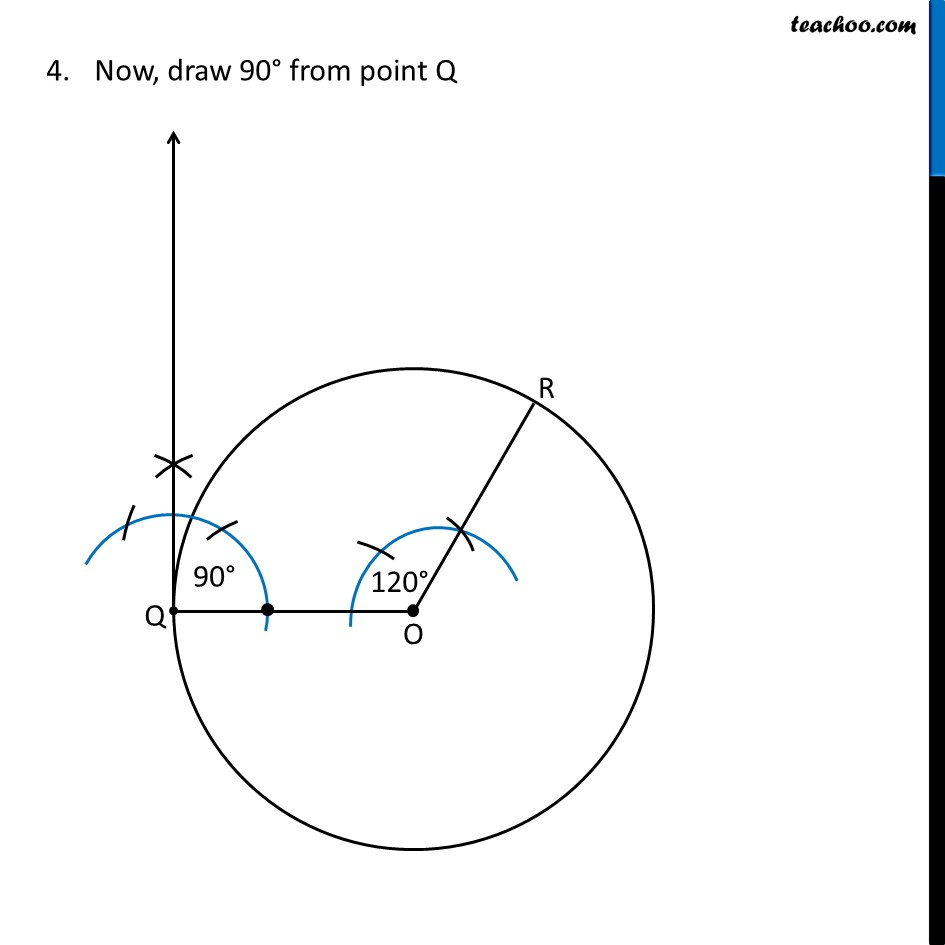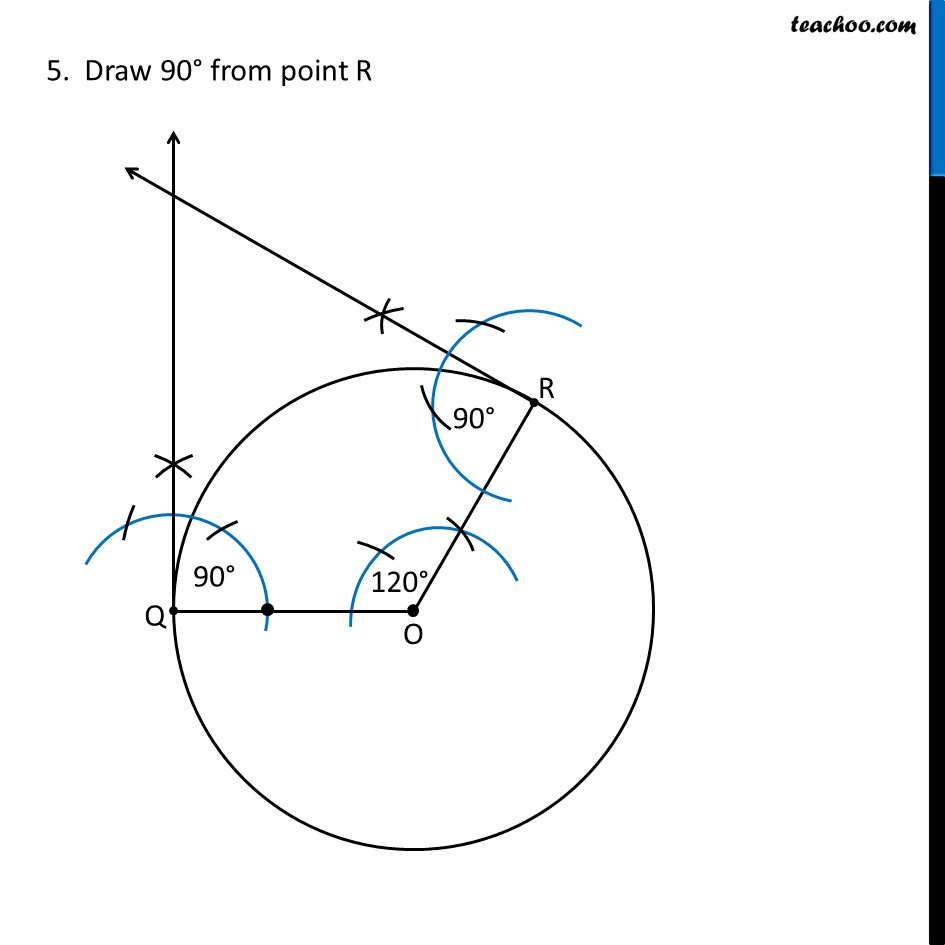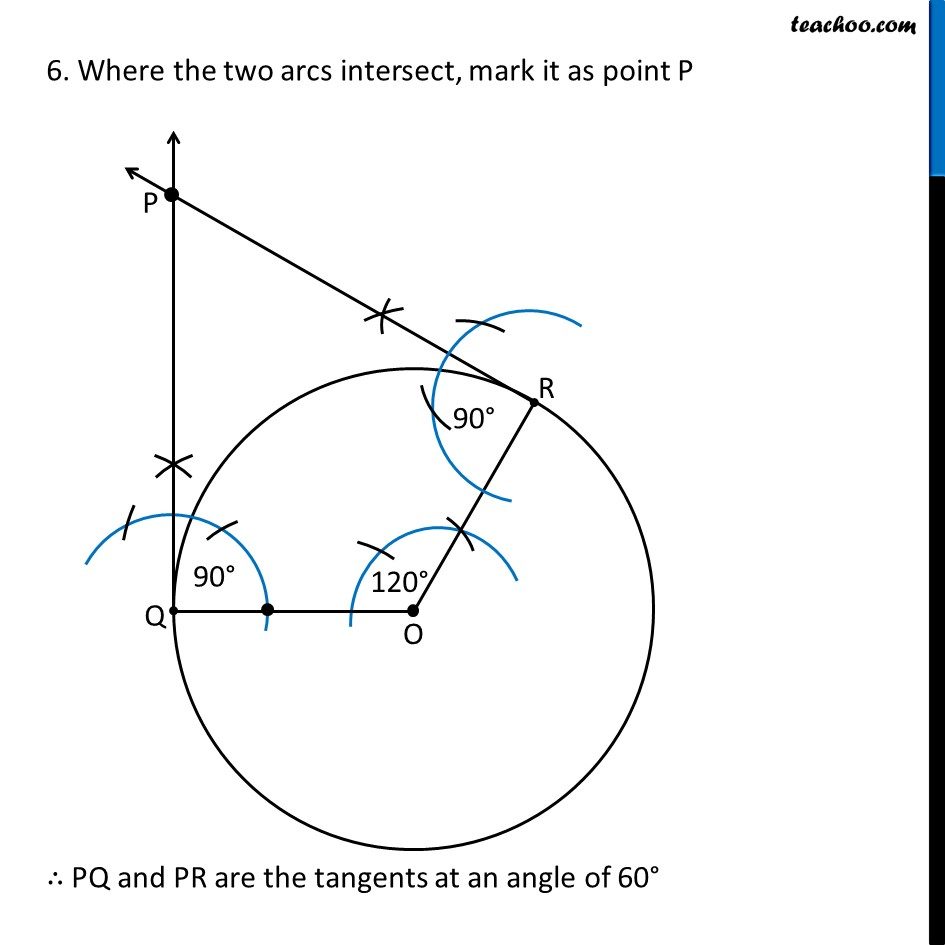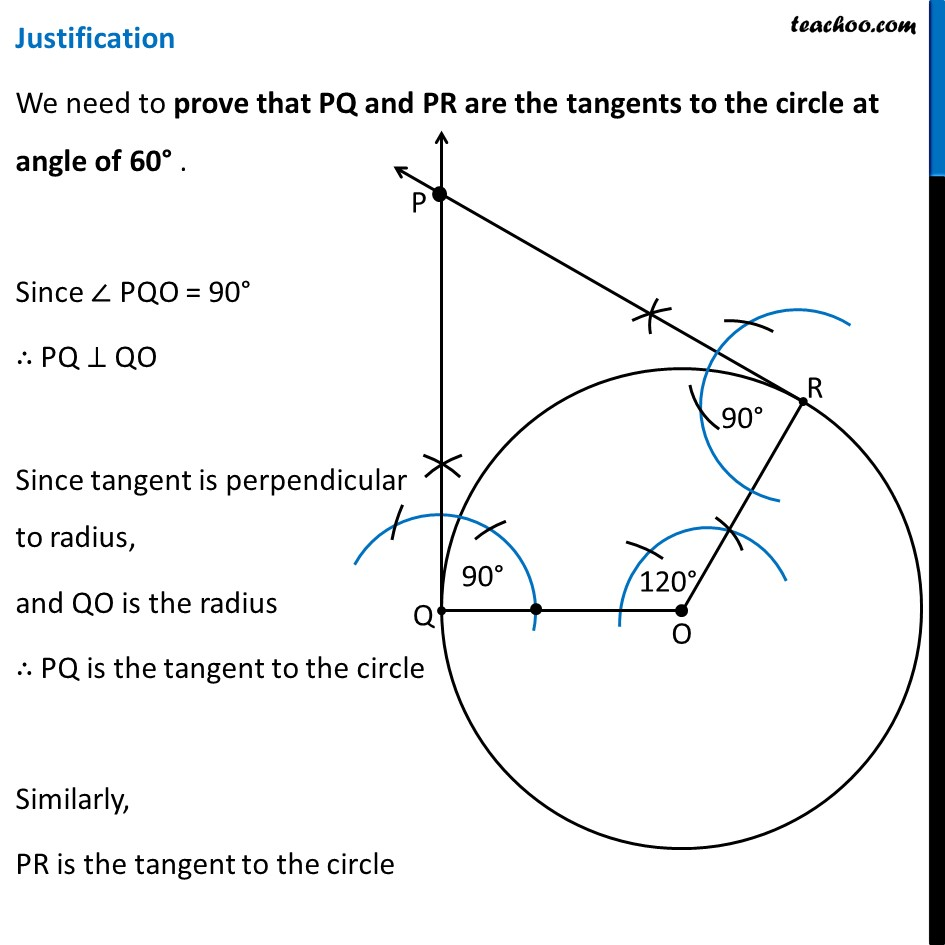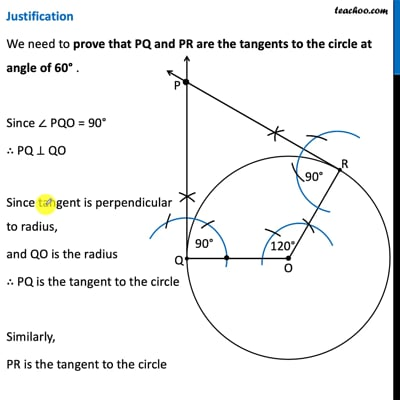This video is only available for Teachoo black users

Maths Crash Course - Live lectures + all videos + Real time Doubt solving!

### Transcript

Ex 11.2, 4 (Concept) Draw a pair of tangents to a circle of radius 5 cm which are inclined to each other at an angle of 60°. Given angle between tangents is 60° i.e. ∠ QPR = 60° Since Angle at center is double the angle between tangents ∴ ∠ OQR = 2 × 60° = 120° So, we need to draw ∠ QOR = 120° ∴ We draw a radius, then second radius at 120° from first. Also, Tangent is perpendicular to radius So, OQ ⊥ QP & OR ⊥ PR Thus, to make tangents, we draw perpendicular from point Q and R So, we draw 90° from point Q and point R Thus, our figure will look like Ex 11.2, 4 Draw a pair of tangents to a circle of radius 5 cm which are inclined to each other at an angle of 60°. Steps of construction Draw a circle of radius 5 cm Draw horizontal radius OQ 3. Draw angle 120° from point O Let the ray of angle intersect the circle at point R Now, draw 90° from point Q 5. Draw 90° from point R 6. Where the two arcs intersect, mark it as point P ∴ PQ and PR are the tangents at an angle of 60° Justification We need to prove that PQ and PR are the tangents to the circle at angle of 60° . Since ∠ PQO = 90° ∴ PQ ⊥ QO Since tangent is perpendicular to radius, and QO is the radius ∴ PQ is the tangent to the circle Similarly, PR is the tangent to the circle Now, we prove ∠ P = 60° In quadrilateral PQOR Sum of angles = 360° ∠ P + ∠ Q + ∠ R + ∠ QOR = 360° ∠ P + 90° + 90° + 120° = 360° ∠ P + 180° + 120° = 360° ∠ P + 300° = 360° ∠ P = 360° – 300° ∠ P = 60° So, PQ and PR are tangents at an angle of 60°